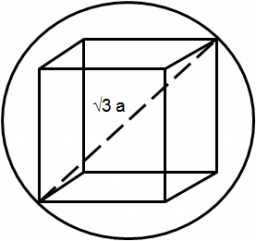# Alien ship

The alien ship has the shape of a sphere with a radius of r = 3000m, and its crew needs the ship to carry the collected research material in a cuboid box with a square base. Determine the length of the base and (and height h) so that the box has the largest possible volume.

a =  4835.9759 m

### Step-by-step explanation:Did you find an error or inaccuracy? Feel free to write us. Thank you!Tips to related online calculators
Tip: Our volume units converter will help you with the conversion of volume units.

#### You need to know the following knowledge to solve this word math problem:

We encourage you to watch this tutorial video on this math problem:

## Related math problems and questions:

• Rectangle poolFind dimensions of an open pool with a square bottom with a capacity of 32 m3 to have painted/bricked walls with the least amount of material.
• Secret treasureScouts have a tent in the shape of a regular quadrilateral pyramid with a side of the base 4 m and a height of 3 m. Find the container's radius r (and height h) so that they can hide the largest possible treasure.
• Regular square prismThe volume of a regular square prism is 192 cm3. The size of its base edge and the body height is 1: 3. Calculate the surface of the prism.
• Prism basesVolume perpendicular quadrilateral prism is 360 cm3. The edges of the base and height of the prism are in the ratio 5:4:2. Determine the area of the base and walls of the prism.
• BallsPing pong balls have a diameter of approximately 5.1 cm. It sold in boxes of 10 pieces: each box has a cuboid shape with a square base. The balls touch the walls of the box. Calculate what portion of the internal volume of the box is filled with balls.
• Prism XThe prism with the edges of the lengths x cm, 2x cm, and 3x cm has volume 20250 cm3. What is the area of the surface of the prism?
• Cube in a sphereThe cube is inscribed in a sphere with a volume 7253 cm3. Determine the length of the edges of a cube.
• Cube-shaped boxThe cube-shaped box is filled to the brim with 2 liters of milk. Calculate the edge and surface of the box.
• Triangular prismThe triangular prism has a base in the shape of a right triangle, the legs of which is 9 cm and 40 cm long. The height of the prism is 20 cm. What is its volume cm3? And the surface cm2?
• Diagonals of a prismThe base of the square prism is a rectangle with dimensions of 3 dm and 4 dm. The height of the prism is 1 m. Find out the angle between the body diagonal with the diagonal of the base.
• Concrete blockDetermine the volume of concrete block whose one edge of the base has a length 3 meters, body diagonal is 13 meters and its height is 12 meters.
• Four prismsQuestion No. 1: The prism has the dimensions a = 2.5 cm, b = 100 mm, c = 12 cm. What is its volume? a) 3000 cm2 b) 300 cm2 c) 3000 cm3 d) 300 cm3 Question No.2: The prism base is a rhombus with a side length of 30 cm and a height of 27 cm. The height of t
• CubesOne cube is an inscribed sphere and the other one described. Calculate the difference of volumes of cubes, if the difference of surfaces in 257 mm2.
• Cube in ballCube is inscribed into the sphere of radius 181 dm. How many percent is the volume of cube of the volume of the sphere?
• Tetrahedral prismThe height of a regular tetrahedral prism is three times greater than the length of the base edge. Calculate the length of the base edge, if you know that the prism volume is 2187 cm3.
• Surface and volume od cuboidContent area of the square base of cuboid is Sp = 36 cm2 and its height 80 mm. Determine its surface area and volume.
• Hollow sphereThe steel hollow sphere floats on the water plunged into half its volume. Determine the outer radius of the sphere and wall thickness, if you know that the weight of the sphere is 0.5 kg and the density of steel is 7850 kg/m3